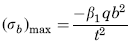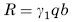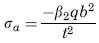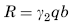Related Resources: calculators

Flat Rectangular Plate, Three Edges Fixed, One Edge (a) Free Loading Uniformly decreasing from fixed edge to zero at 1/3b Equation and Calculator.

Per. Roarks Formulas for Stress and Strain for flat plates with straight boundaries and constant thickness

Flat Rectangular plate, Three Edges Fixed, One Edge Loading Uniformly decreasing from fixed edge to zero at at 1/3b.

 Rectangular plate; Three Edges Fixed One Edge FreeLoading Uniformly decreasing from fixed edge to zero at at 1/3bAt x = 0, z = 0
Stress and Reaction ForceReaction ForceStress and Reaction Force at x = ± a/2, z = 0.2bReaction ForceWhere used:
E = Modulus of Elasticity (lbs/in2)
q = Total load or force to Plate (lbs/in2)
v = Poisson’s ratio (assumed to be 0.3)
t = plate thickness, (in)
a = plate length, (in)
b = plate width, (in)
σb = stress, (lbs/in2)
R = Reaction Force (lbs/in)
β1,2 = Constant From Table A
γ1,2 = Constant From Table A

Table A

 a/b 0.25 0.5 0.75 1 1.5 2 3 β1 0.014 0.035 0.047 0.061 0.076 0.086 0.1 β2 0.01 0.024 0.031 0.03 0.025 0.02 0.014 γ1 0.088 0.13 0.146 0.156 0.162 0.165 0.167 γ2 0.046 0.069 0.079 0.077 0.073 0.073 0.079

Reference:

Roarks Formulas for Stress and Strain, 7th Edition